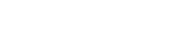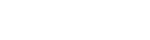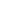AlmaBetter Blogs > Introduction to Machine Learning

# Introduction to Machine Learning

Data Science
The above picture shows us how far we have come in the field of information technology. Since the inception of the first computer designed by Charles Babbage in the early nineteenth century, we have come a long way.The above picture shows us how far we have come in the field of information technology. Since the inception of the first computer designed by Charles Babbage in the early nineteenth century, we have come a long way. Today, Computers are not just smaller but smarter as well. In recent decades, we have reached a breakthrough in the field of computer science with astonishing advancements in the field of transmitting human intelligence to computers.

Today, we have machines that can mimic human behavior and make predictions by understanding the pattern of thousands of terabytes of information (popularly known as Big Data). You may come across various terminologies to describe the recent developments such as AI, Data Science, Machine Learning, and Deep Learning. But let’s not get ahead of ourselves. Let’s start from the basics and understand each term individually.

1. Artificial Intelligence (AI): Artificial Intelligence is an umbrella term used to define all the technological advancements and algorithms that enable machines to demonstrate human intelligence.

2. Machine Learning (ML): Machine Learning is a subset of artificial intelligence. ML involves learning the pattern of real-life scenarios from the input data based upon certain algorithms to provide accurate predictions.

3. Deep Learning: Deep learning is a subset of Machine Learning which is concerned with algorithms that mimic the workings of a human brain. These algorithms which have similar structure and function to a human brain are popularly known as Neural Networks.You can understand the relationship between AI, ML, and Deep Learning from the diagram shown below:-

Since we have covered the basic terminologies, let’s deep dive into the workings of Machine Learning.

## What is Machine Learning?

In layman's terms, machine learning involves the use of algorithms to make the machine learn on its own from a given input dataset. In other words, it involves the study of those computer programs which automatically improve their performance through experience.

The Term Machine Learning was first coined by Arther Samuel in 1952. He described ML in the following words.

“Machine learning is the practice of giving computers the ability to learn without being explicitly programmed to do so.”

After Arther, a more formal and widely popular definition of Machine Learning was propounded by Tom Mitchell. Let us get a wider perspective about Machine Learning from his words mentioned below.

“A computer program is said to learn from experience E with respect to some class of tasks T and performance measure P if its performance at tasks in T, as measured by P, improves with experience E.”

Now let us understand the meaning of the ETP definition of ML by viewing the examples mentioned in the purple boxes below.## Tasks of Machine Learning

Supervised Learning

It is defined by its use of labeled datasets to train ML algorithms in order to classify data or predict outcomes accurately. As input data is entered into the model, it adjusts its parameters until we get a fitted model that appropriately translates the relationship between the independent and dependent variables. Some of the techniques used in supervised Machine Learning include linear regression, logistic regression, neural networks, and naïve Bayes.

There can be two instances while undergoing supervised learning, namely:-

1. Regression: We define a supervised ML problem as a regression problem if the dependent variable or the target variable is continuous in nature. For instance forecasting, the weather report of a city-based upon technical factors such as wind speed, humidity levels, seasonal variations, etc can be an example of a regression problem.

2. Classification: We define a supervised ML problem as a Classification problem if the dependent variable or the target variable is categorical in nature. For example: if a bank wants to analyze whether to give a loan to a particular account holder based upon CIBIL score, account balance, past loans repayment, etc. This is an example of a classification problem since our dependent variable can only take two values, YES (the bank will accept the loan application) and NO (the bank will reject the loan application).Unsupervised Machine Learning

When the target variable is absent from the initial dataset then we use Unsupervised Machine Learning. Since we don’t have a target set of values to supervise our model’s working, we use this ML technique to analyze and cluster unlabeled datasets. Hereby Cluster, we mean the formation of segments based upon the pattern of the input dataset. Cluster analysis finds the common points or similarities between the data objects and categorizes them as per the presence and absence of those common characteristics. Some of the most commonly used ML algorithms in Unsupervised scenarios include k-means clustering, neural networks, Principle Component Analysis, and probabilistic clustering methods.

For example, clustering is a very commonly used technique to build recommendation systems for OTT, finding customer segments for airline loyalty programs from a given dataset of air passengers by Delta Airlines, and much more.Reinforcement Learning

Reinforcement machine learning is a behavioral machine learning model that is similar to supervised learning, but the algorithm isn’t trained using sample data. This model learns by using a trial and error approach while working in an environment. The model’s output is assessed by the environment and the outcomes are labeled as desired and undesired. The desired outcomes are rewarded so the algorithm tries to maintain a streak of the desired outcomes consecutively. Therefore, a sequence of successful outcomes will be reinforced by the environment to develop the best recommendation or policy for a given problem statement.

For example, the IBM Watson system competed against the previous champions of Jeopardy! the challenge in 2011 and won by using this form of ML algorithm.

This was all for today. We’ll discuss more about the various techniques of Machine learning in future blogs. so stay tuned and like this article, if you gained some clarity on machine learning.

References

1. Machine-learning mastery articles.

2. wikimedia.com

3. zldoty article on what is machine learning.

4. IBM machine learning article.

5. Alma Better Course Materials.## Related PostsMade within Bengaluru, India